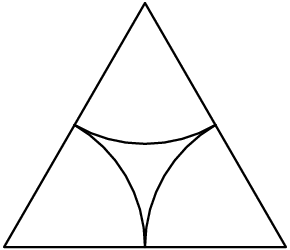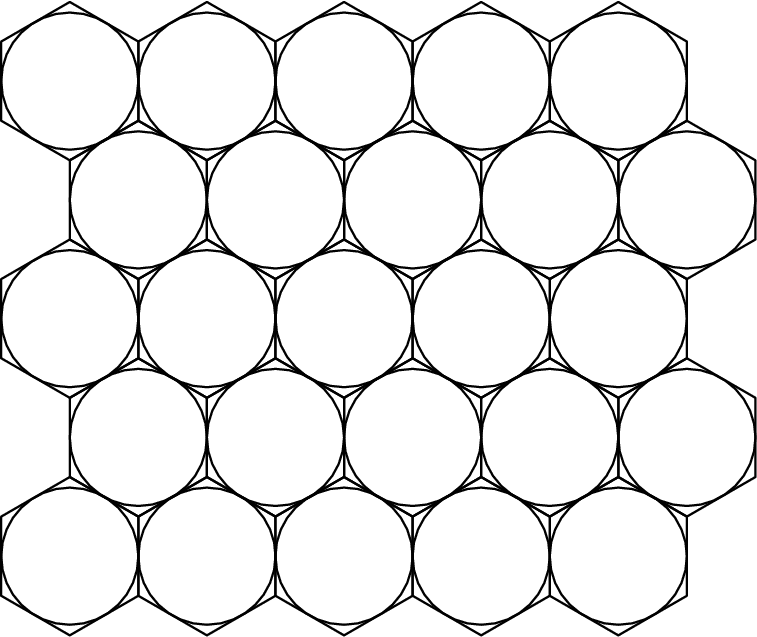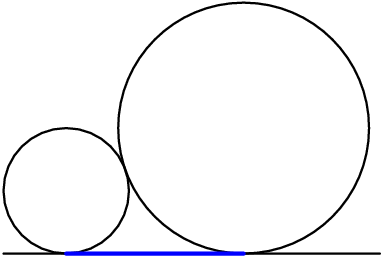# Why does Prof. Loh use all these triangles? Why not something else?

• Module 2 Week 3 Day 9 Your Turn Explanation Part 1

How do you know that they are congruent if it is not stated?

• Hi bravekiwi,

If you are referring to the cookies, or the circles, in the diagram, you may assume they are congruent because cookie machines normally make cookies of the same size. You are correct that if we were being as precise as possible, we would say this. But it's safe to assume that the cookie machine makes cookies of a uniform size.

Happy Learning,

The Daily Challenge Team

• Why does Prof. Loh use all these triangles? Why not something else?

• And towards the end, why did Prof. Loh divide and not subtract?

• Hi again!

These are great questions! There are actually many ways to go about solving this question. In particular, there are multiple ways to "dissect" that whole sheet of cookies into simpler shapes.

The idea behind "dissecting" this cookie sheet was to find a single shape that can cover the whole sheet, and so that the "pattern" inside every shape is the same. Professor Loh decided to use equilateral triangles by connecting the centers of the circles, and each triangle had this same pattern inside:If each triangle has the same "pattern" inside, then each triangle has the same amount of cookie inside! So by just looking at one triangle, you can analyze the whole sheet of cookies because the rest of the triangles are the same!

There are more ways to do it though! For example, maybe we can use hexagons:This also works! Can you try to work it out?

I think the first dissection using triangles is a little easier. One important reason is this: When circles are tangent to each other, it is usually very useful to draw a line connecting their centers! It's almost always a very helpful first step in any geometry problem. For example, how would you find the length of this blue line segment if the radii of the circles are 1 and 2?(Try connecting the centers!)

As for your other question: Professor Loh divides because he wants to find the fraction of the triangle that is taken up by the circles (the "cookies"). By doing this division, he can actually figure out what fraction of the ENTIRE sheet is covered by circles! If he only subtracted, he'd only find the area within one triangle taken up by cookies, and that's not enough.

Let me know if you have any other questions!